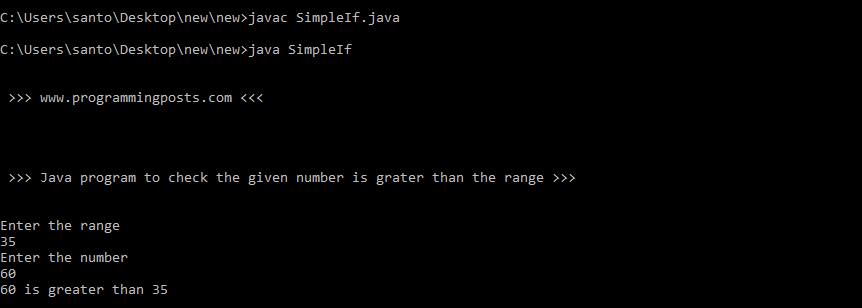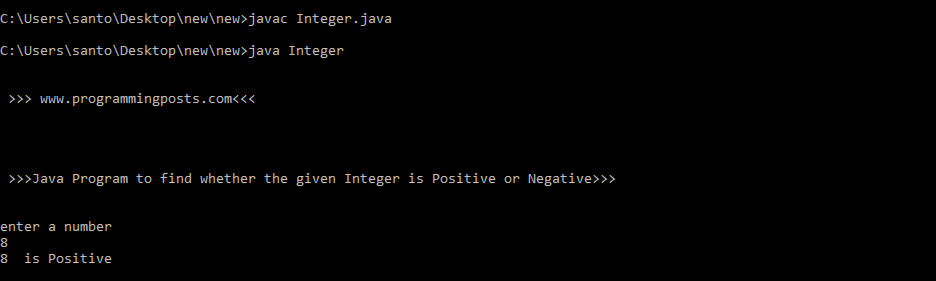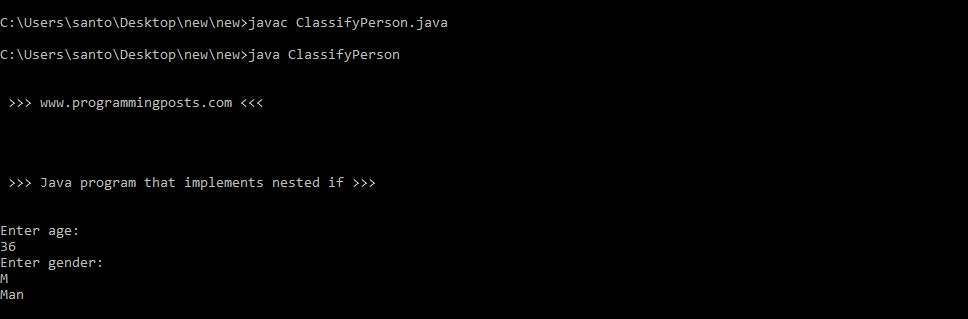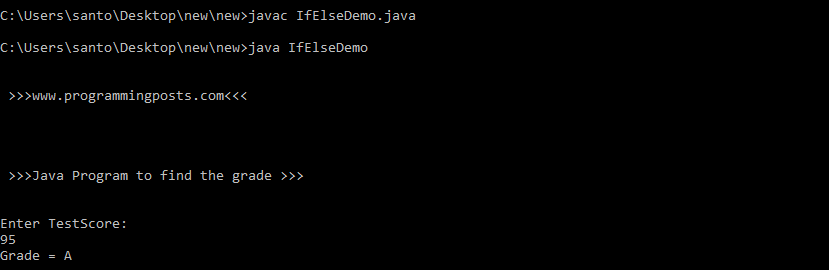# Java program to implement simple if-else

In programming, we execute certain section of code based on whether the specified condition is true or false which is known only at run time. For such cases we use “control flow statements”.

• Simple if
• if……else
• nested if
• if else if

### Simple if:

Simple If statement is the most basic of all the control flow statements. It tells your program to execute a certain section of code only if a particular test evaluates to true.

### Syntax:

if(condition){

//code to be executed

}

if the condition in if statement is true then it executes the code in if block. if not true it skips the code in the block and executes the rest of the code.

Lets see an example for it.

in this example we check the given number is greater than the declared number.

### Algorithm:

Step 1 : START.Step 2 : Read two numbers, say range, num.

Step 3 : check the eligibility using range>num.

Step 4 : Print the result.

Step 5 : STOP.

### Source code:

```import java.util.Scanner;
class SimpleIf
{
public static void main(String args[])
{
System.out.println("\n\n >>> www.programmingposts.com <<< \n\n");
System.out.println("\n\n >>> Java program to check the given number is grater than the range >>> \n\n");
Scanner scanner = new Scanner(System.in);
System.out.println("Enter the range");
int range= scanner.nextInt();
System.out.println("Enter the number");
int num= scanner.nextInt();
if(num>range)
{
System.out.println(num + " is greater than " + range);
}
}
}
```

### Explanation:

The user is asked to enter two numbers. One is the number(num) and the other is the number(range) with which num is to be compared. Then we check the condition given in the if statement for true. if it is true it displays the statement that the given number(num) is greater than the range.

### Output:

C:\Users\santo\Desktop\new\new>javac SimpleIf.java

C:\Users\santo\Desktop\new\new>java SimpleIf

>>> www.programmingposts.com <<<

>>> Java program to check the given number is grater than the range >>>

Enter the range
35
Enter the number
60
60 is greater than 35

### Screenshot of output:Screenshot of output

### if….else:

The if…else statement executes some code if the test expression is true (nonzero) and some other code if the test expression is false (0).

### Syntax:

if(condition){

//code  for if

}

else{

//code for else

}

if condition is true, code inside the body of  if  statement is executed and, code inside the body of else statement is skipped.

if condition is false, code inside the body of if statement is skipped and, code inside the body of else statement is executed.

Lets see an example that implements If-else statement.

Java Program to find the given Integer is Positive or Negative

### Algorithm:

Step 1 : START.

Step 2 : Read an Integer, say n.

Step 3 : if n>0 then n is Positive Integer.

Step 4 :  else n is negative number.

Step 5 : STOP.

### Source code:

```import java.util.*;
class Integer
{
public static void main(String args[])
{
System.out.println("\n \n >>> www.programmingposts.com<<< \n \n");
System.out.println("\n\n >>>Java Program to find whether the given Integer is Positive or Negative>>> \n \n ");
System.out.println("enter a number");
Scanner scanner = new Scanner(System.in);
int num = scanner.nextInt();
if(num>0)
{
System.out.println(num + "  is Positive");
}
else
{
System.out.println(num + " is negative");
}
}
}```

### Explanation:

An Integer is said to be Positive Integer, if it is greater than Zero(0). else it is said to be negative Integer.

The user is asked to enter a number and is stored in a variable(num) of Integer data type. Now we check the number that is greater than Zero(0) or not using IF…. ELSE Statement. if the number is greater than Zero then the given number is Positive else it is negative.

### Output:

C:\Users\santo\Desktop\new\new>javac Integer.javaC:\Users\santo\Desktop\new\new>java Integer

>>> www.programmingposts.com<<<

>>>Java Program to find whether the given Integer is Positive or Negative>>>

enter a number
8
8 is Positive

### Screenshot of output:Screenshot of output

### nested if:

nested if means we can use one if  or else if statement inside another if or else if statement.

### Syntax:

if(condition){

if(condition)

{

//code for if

}

}

Let us see an example which implements nested if.

```import java.util.Scanner;
class ClassifyPerson
{
public static void main(String args[])
{
System.out.println("\n\n >>> www.programmingposts.com <<< \n\n");
System.out.println("\n\n >>> Java program that implements nested if >>>\n \n");
Scanner scanner = new Scanner(System.in);
System.out.println("Enter age:");
int age = scanner.nextInt();
System.out.println("Enter gender:");
char gender = scanner.next().charAt(0);
if(age > 35)
{
if( gender == 'M')
{
System.out.println("Man");
}
else
{
System.out.println("Woman");
}
}
else
{
if( gender == 'M')
{
System.out.println("Boy");
}
else
{
System.out.println("Girl");
}
}
}
}```

### Explanation:

In the above program depending on the age we classify the person based on his age. So, we have taken two variable age and gender which are of int and char datatype.  if the age is greater than 35 then it executes the inner loop. or it e executes the inner loop of else statement.

for example let us consider age= 34 and gender = ‘F’ first it checks the if loop whether the condition is true or not. the condition is false. So it executes else part. Then it checks for gender is M or F. In the else part the inner if statement is checked for the condition is true or not. Here the gender is F so it executes else part of the else loop.

### Output:

C:\Users\santo\Desktop\new\new>javac ClassifyPerson.javaC:\Users\santo\Desktop\new\new>java ClassifyPerson

>>> www.programmingposts.com <<<

>>> Java program that implements nested if >>>

Enter age:
36
Enter gender:
M
Man

### Screenshot of Output:Screenshot of output

### if -else-if:

Java control flow statements are executed from top to down, therefore, a ladder of if-else conditions will be evaluated from top to down. As soon as an if statement from the ladder evaluates to true, the statements associated with that if are executed, and the remaining part of the ladder is bypassed. The last most else is executed only when no condition in the whole ladder returns true.

### Syntax:

if(condition1){//code to be executed if condition1 is true

}

else if(condition2){

//code to be executed if condition2 is true

}

else if(condition3){

//code to be executed if condition3 is true

}

else{

//code to be executed if all the conditions are false

}

Lets see an example program that implements if-else-if  statement.

Java program to find the grade based on the test score that the student scored in an examination.

### Source code:

```import java.util.*;
class IfElseDemo
{
public static void main(String args[])
{
System.out.println("\n\n >>>www.programmingposts.com<<< \n\n");
System.out.println("\n\n >>>Java Program to find the grade >>>\n\n");
System.out.println("Enter TestScore:");
Scanner scanner = new Scanner(System.in);
int testscore = scanner.nextInt();
char grade;
if (testscore >= 90)
{
grade = 'A';
}
else if (testscore >= 80)
{
grade = 'B';
}
else if (testscore >= 70)
{
grade = 'C';
}
else if (testscore >= 60)
{
grade = 'D';
}
else
{
grade = 'F';
}
System.out.println("Grade = " + grade);
}
}```

### Explanation:

In the above program the user is asked to enter the test score that he scored in an examination. That test score is stored in a variable of integer datatype say testscore. The testscore is compared with in the limits of each grade. if both the testscore and the limit of grade are equal then that grade is awarded.

### Output:

C:\Users\santo\Desktop\new\new>javac IfElseDemo.javaC:\Users\santo\Desktop\new\new>java IfElseDemo

>>>www.programmingposts.com<<<

>>>Java Program to find the grade >>>

Enter TestScore:
95
Grade = A

Screenshot of Output:Screenshot of output